Next: PROPERTIES OF THE GF Up: What is Green's Function Previous: HEAT EQUATION EXAMPLE

# Another Interpretation of G

There is a second physical interpretation of the GF for transient problems, as follows: the GF may also be considered as the temperature response to a concentrated initial condition. Consider the following boundary value problem: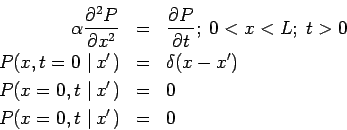The above equations are nearly identical to the equations defining G, if the time at which the heat source is released has been set to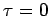. Barton (1989) calls function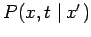the propagator; it's relation to the GF is given explicitly by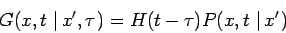where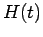is the Heaviside unit-step function. Several GF in this Library have been found with this view point.

2004-01-21